The riprap equation has several empirical coefficients or coefficient equations that the riprap calculator selects based on user options in the Edit ribbon: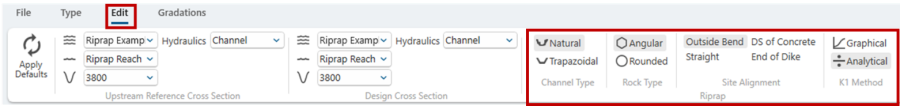These options translate into the following equation coefficients, equations, and parameters in the d30 calculation described in the Step 4: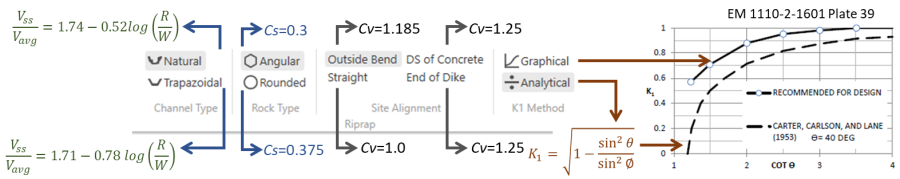These parameters and equations will be discussed in more detail in the next section on the d30 calculations, but are briefly described below:

Channel Type:
Choose Natural for a non-engineered channel with native materials in the bed and banks and some bank vegetation. Chose Trapezoidal for an engineered channel. The riprap calculator will choose the equation to compute the side slope velocity based on this selection. The calculator will generally compute higher side slope velocities for trapezoidal channels for all observed bends (as long as R/W>1).

Rock Type: The Stability Coefficient (Cs) was the original coefficient in the riprap equation. This is a linear coefficient in the equation, and is 0.3 for rounded rock (e.g. natural river stone and cobbles) and 0.375 for angular rock (e.g. riprap).Warning

Community partners, project biologists, and landscape architects will often advocate for rounded river rock instead of angular, crushed riprap because it is aesthetically appealing. It is important to carefully account for rock type in the stability analysis. According to this equation, rounded rock must be 25% larger and 100% heavier (weight increases to the cube power of diameter) to provide comparable protection.

Site Alignment
: The riprap calculator will also require a larger d30 if the site alignment deviates from a straight channel. This option selects the Vertical Velocity Distribution Coefficient (Cv) in the riprap equation, which is 1 for straight channels and 1.25 for locations where the channel transitions Images?
from an engineered section to an un-protected section (downstream of a concrete lining or the end of a dike. At the outside of the bend, it computes the Cv coefficient based on the logarithm of the R/W ratio: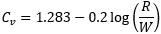This value falls between 1 and 1.22 for 2<R/W<25. If R/W>25 the equation computes Cv less than 1, but the Riprap Calculator enforces a minimum value of Cv=1.

K1 Method: The riprap equation incudes a Side Slope Correction Factor (K1). This factor is in the denominator of the Maynord equation and is a function of a trigonometric function of the side slope angle (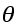), making the rock size directly related side slope (e.g. steeper slopes require larger rock).

Early approaches used a relationship between the side slope angle and the angle of repose from Carter, Carleson, and Lane (1953):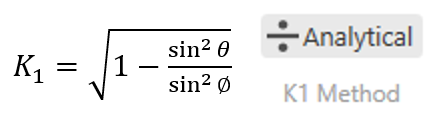This is the "Analytical Approach" in the Riprap Calculator.

But the USACE Manual of Practice (EM 1110-2-1601) follows Maynord (1988), who found this relationship too conservative, and unhelpfully dependent on the angle of repose – which is uncertain. Therefore, the USACE recommended approach selects K1 based on a recommended curve from Plate 39 in EM 1110-2-1601: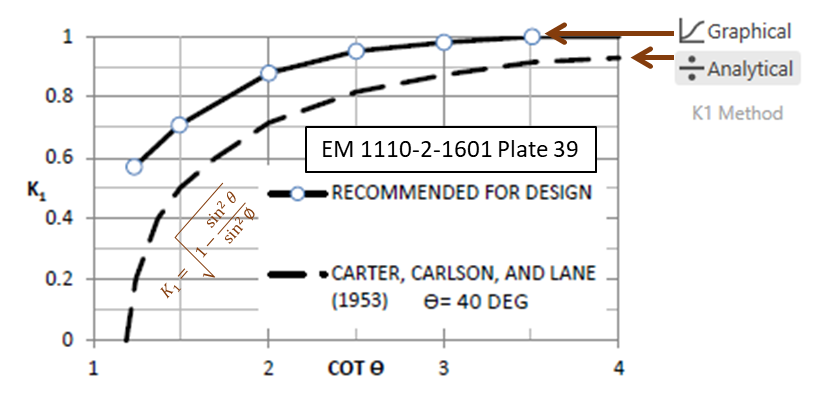This is the "Graphical Approach" in the Riprap Calculator, which is the default.Note:

Increasing the side slope has a direct, gravitational, impact on side slope rock size. But the K1 factor is also included in the bed size equation. This effect is less direct.Note: Slope Angle Approaching Angle of Repose

In some riprap sizing methods, as the side slope approaches the angle of repose, the required rock size approaches infinity because the rock is at the critical conditions for incipient motion.